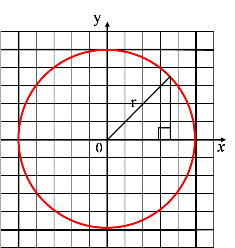The circle is a plane curve. It is a conic section.

The circle is defined as the path of a point (locus) which moves such that its distance (called the radius) from a fixed point (called the centre) is a constant.

When the centre is (0,0) and the radius is r the equation of the circle in Cartesian Coordinates is $x^2+y^2=r^2$

This is closely related to the Pythagoras' Theorem.

One of the Named Curves on this site.